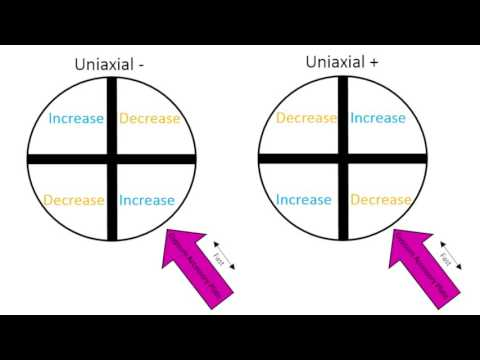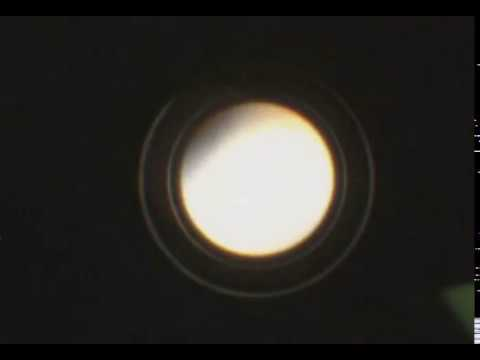# 2.8: Interference Figures

Interference figures are a technique which can be used to help identify minerals using a polarizing light microscope. In this chapter, we explore the practical aspects of obtaining and interpreting interference figures and other related observations.

Learning Objectives

Students should be able to:

• Describe the steps used to obtain interference figures.
• Correctly name the parts of an interference figure.
• Describe the features of an indicatrix.
• Identify the type of interference figure produced.
• Connect crystal symmetry to the type of interference figure(s) that are produced.
• Use interference figures to narrow down the possible symmetry choices and help identify a mineral.
• Make optical sign determinations and 2V angle estimates to further help identify minerals (optional).

## How to Obtain an Interference Figure

These videos show the steps to obtaining an interference figure. They are shot at two different angles so it is possible to see the operation of the microscope better.A YouTube element has been excluded from this version of the text. You can view it online here: pb.libretexts.org/petrology/?p=112A YouTube element has been excluded from this version of the text. You can view it online here: pb.libretexts.org/petrology/?p=112

Figure 2.8.1 A and 2.8.1 B. Basic steps to obtain an interference figure on a mineral.

In each of these videos, there is a single mineral crystal on the slide. If you are looking at multiple grains of the same mineral, you may find it helpful tolocate mineral grains with the lowest interference colors (lowest retardation) to obtain interference figures that are the easiest to identify (e.g., uniaxial optic axes figures, or biaxial acute bisectrix figures, which will be described below).

Guided Inquiry

The original version of this chapter contained H5P content. You may want to remove or replace this element.

Question 2.8.1. Drag and drop the steps of obtaining an interference figure in the correct order.

## Anatomy of an Interference Figure

Below is an example of one type of interference figure. This figure contains three types of features: isogyres, a melatope, and isochromes.

The original version of this chapter contained H5P content. You may want to remove or replace this element.

Figure 2.8.3. Anatomy of an interference figure.

## The Indicatrix

The index of refraction is different in different crystallographic directions within a mineral, according to its crystal symmetry. We can characterize this using an ellipsoid (roughly the shape of an American football or rugby ball-shaped object) which shows the refractive index in every direction, including the three principle optical axes. This ellipsoid is called an indicatrix.

The indicatrix is a 3-dimensional visualization of the indices of refraction of a mineral. As discussed previously in light and optics, the index of refraction is different for different minerals. It can also vary as a function of direction within the crystal structure. Minerals with lower symmetries have greater variation in the index of refraction with direction.Figure 2.8.4. Examples of a uniaxial indicatrix and a biaxial indicatrix. The indices of refraction are plotted parallel to the vibration direction of light.

As discussed in Section 2.3 Light and Optics, Birefringence, a solid material such as a mineral can split light waves into two polarized beams, the ordinary ray (represented by “o” or “ω” and the extraordinary ray (represented by “e” or “ε”). Uniaxial minerals exhibit the same refractive index along two principal axes (), and a different refractive index along the third principal axis (). Biaxial minerals have different refractive index values along each principal axis, with as the lowest refractive index, as an intermediate refractive index, and as the largest refractive index.

Why create this type of diagram? It is useful for thinking about how the interference figure will vary as the crystal is oriented in different directions in the thin section. Indicatrices are shown in the figures showing uniaxial and biaxial interference figures in the sections below. The figures show the orientation of the indices of refraction with respect to the thin section surface using the indicatrix, and the resulting interference figure.

## Uniaxial Interference Figures

The following two videos explain how to obtain uniaxial interference figures and how to determine optic sign for a uniaxial mineral. Figure 2.8.7 summarizes the types of interference figures that can be observed for uniaxial minerals.A YouTube element has been excluded from this version of the text. You can view it online here: pb.libretexts.org/petrology/?p=112

Figure 2.8.5. Earth Optics Videos. Video 4: Uniaxial Minerals, CC-BY license. https://youtu.be/JShFwEIqUzYA YouTube element has been excluded from this version of the text. You can view it online here: pb.libretexts.org/petrology/?p=112

Figure 2.8.6. Earth Optics Videos. Video 5: Uniaxial Flash Figure, CC-BY license. https://youtu.be/KBwFSC4vPRcFigure 2.8.7. Different types of interference figures produced by uniaxial minerals. For simplicity, isochromes are not shown. The lower part of each image shows the orientation of the indicatrix for the mineral. The middle part of the diagram shows the interference figure relative to the thin section and the upper image shows the view through the microscope ocular.A YouTube element has been excluded from this version of the text. You can view it online here: pb.libretexts.org/petrology/?p=112

Figure 2.8.8. Uniaxial optic axis figure obtained on quartz.A YouTube element has been excluded from this version of the text. You can view it online here: pb.libretexts.org/petrology/?p=112

Figure 2.8.9. Uniaxial flash figure obtained on quartz.

Guided Inquiry

The original version of this chapter contained H5P content. You may want to remove or replace this element.

## Biaxial Interference Figures

The following two videos explain how to obtain biaxial interference figures, how to determine optic sign for a biaxial mineral, and how to estimate a 2V angle.A YouTube element has been excluded from this version of the text. You can view it online here: pb.libretexts.org/petrology/?p=112

Figure 2.8.10. Earth Optics Videos. Video 6: Biaxial Minerals. CC-BY license. https://youtu.be/pA_fJnbPRtgA YouTube element has been excluded from this version of the text. You can view it online here: pb.libretexts.org/petrology/?p=112

Figure 2.8.11. Earth Optics Videos. Video 7: Biaxial Flash Figure. CC-BY license. https://youtu.be/qCkljJ1Isjw

Biaxial interference figure diagrams.Figure 2.8.12.A. Different types of interference figures produced by biaxial minerals. For simplicity, isochromes are not shown. The lower part of each image shows the orientation of the indicatrix for the mineral. The middle part of the diagram shows the interference figure relative to the thin section and the upper image shows the view through the microscope ocular.
M= melatope; Bxa = acute bisectrix; Bxo = obtuse bisectrix.Figure 2.8.12.B. Different types of interference figures produced by biaxial minerals. For simplicity, isochromes are not shown. The lower part of each image shows the orientation of the indicatrix for the mineral. The middle part of the diagram shows the interference figure relative to the thin section and the upper image shows the view through the microscope ocular.
Bxa = acute bisectrix; Bxo = obtuse bisectrix.A YouTube element has been excluded from this version of the text. You can view it online here: pb.libretexts.org/petrology/?p=112

Figure 2.8.13. An acute bisectrix optical interference figure obtained on albite.A YouTube element has been excluded from this version of the text. You can view it online here: pb.libretexts.org/petrology/?p=112

Figure 2.8.14. An optical interference figure obtained on an augite crystal oriented parallel to the 001 crystallographic direction.A YouTube element has been excluded from this version of the text. You can view it online here: pb.libretexts.org/petrology/?p=112

Figure 2.8.15. An optical flash figure obtained on an augite crystal.

Guided Inquiry

The original version of this chapter contained H5P content. You may want to remove or replace this element.

## HomeInterference Figures and Crystal Symmetry

The types of interference figures that a mineral produces are related to the crystal symmetry of that mineral. The figure below summarizes the division of crystal symmetries into isotropic, uniaxial, and biaxial optical properties.Figure 2.8.16. The crystal systems labelled with optical properties (isotropic, uniaxial, or biaxial). If the symmetry of a crystal is known, it can be used to predict which type of interference figures the mineral will produce in thin section.

Guided Inquiry

The original version of this chapter contained H5P content. You may want to remove or replace this element.

## Synthesis: Is it Uniaxial or Biaxial?A YouTube element has been excluded from this version of the text. You can view it online here: pb.libretexts.org/petrology/?p=112

Figure 2.8.17. An interference figure obtained on a calcite crystal.A YouTube element has been excluded from this version of the text. You can view it online here: pb.libretexts.org/petrology/?p=112

Figure 2.8.18. An interference figure obtained on a nepheline crystal.

Guided Inquiry

The original version of this chapter contained H5P content. You may want to remove or replace this element.

The original version of this chapter contained H5P content. You may want to remove or replace this element.